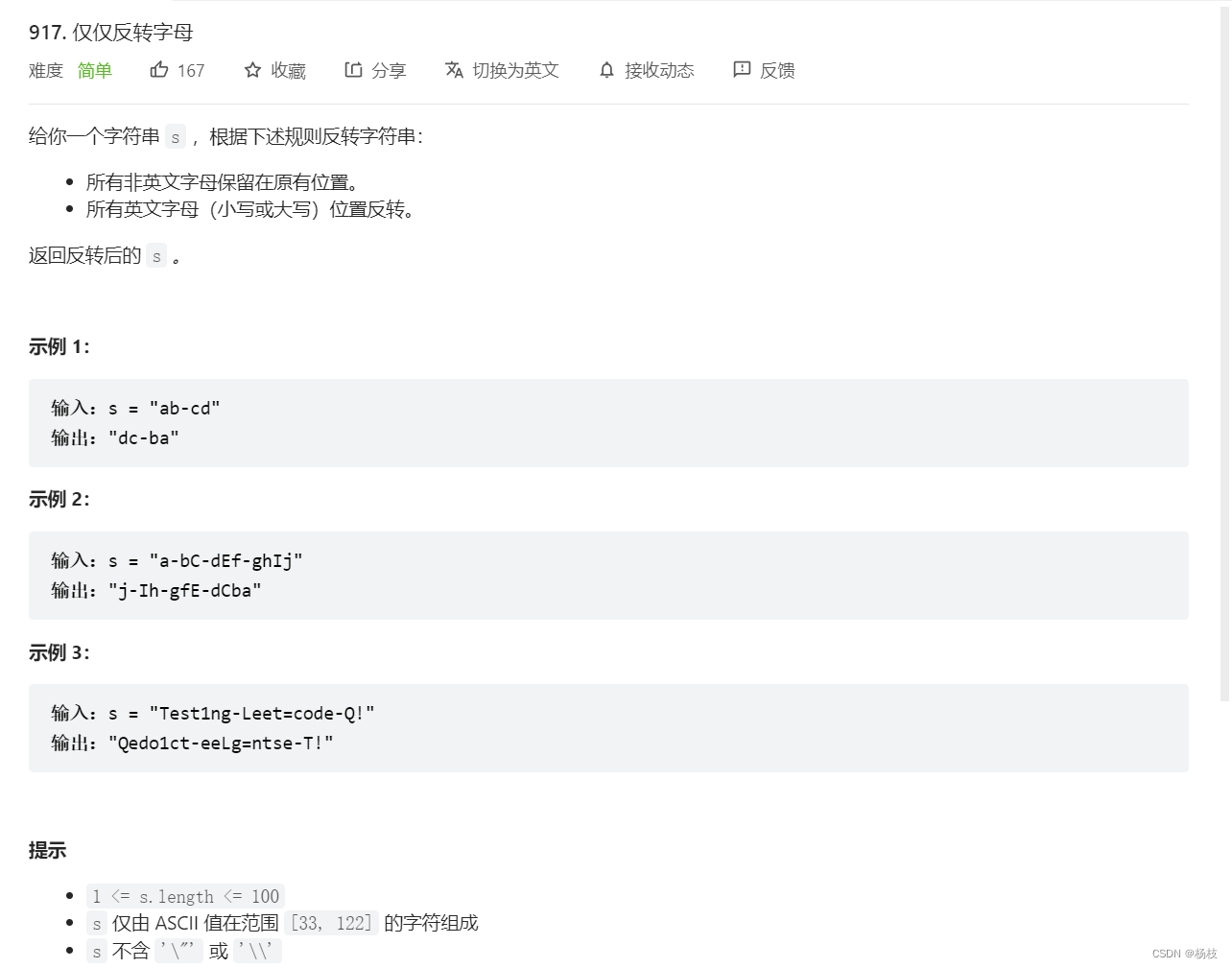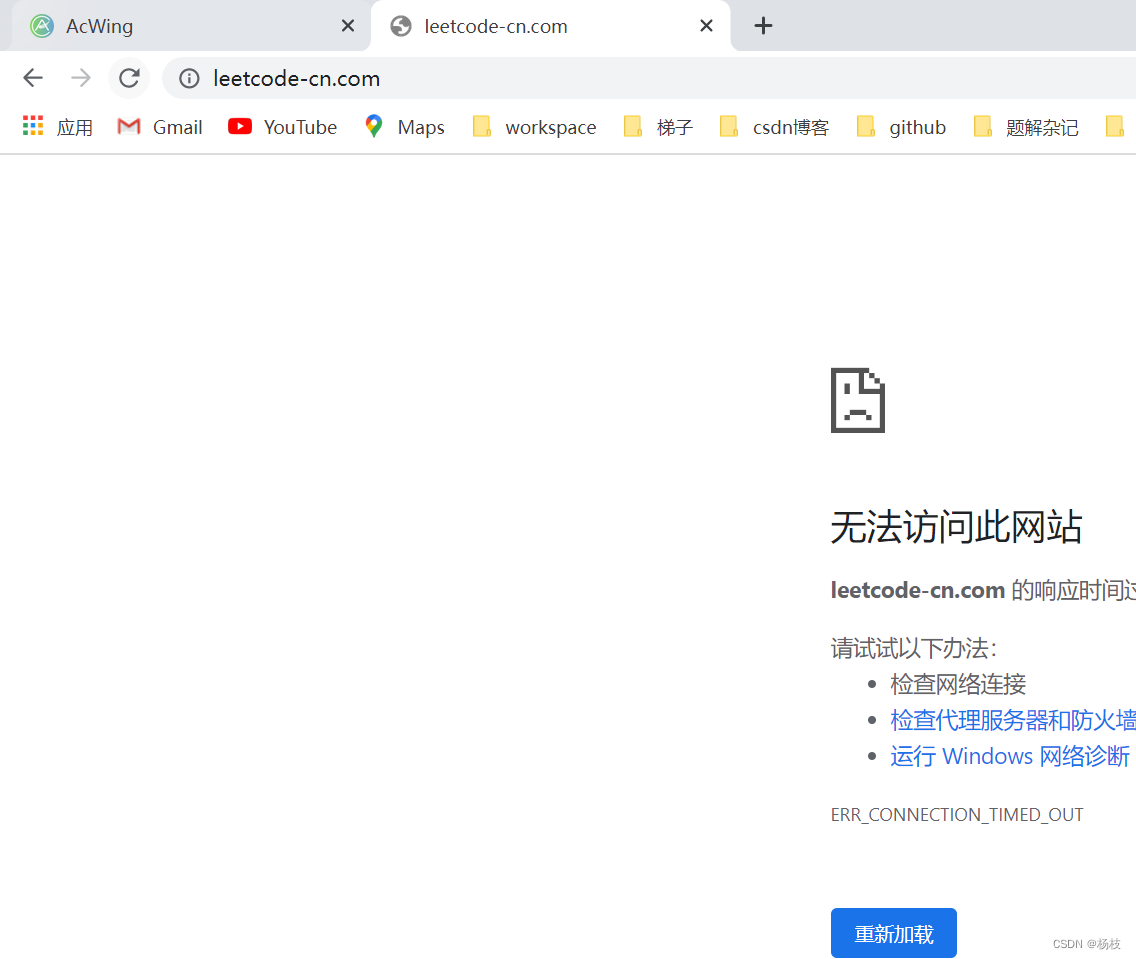﻿• 价格透明
• 信息保密
• 进度掌控
• 售后无忧# 学东西总是像极了暴力枚举的我，想做一枚快乐的双指针

### 目录

• 前言
• 第一题 917. 仅仅反转字母
• 💒题目描述
• 🌟解题报告
• 🌻参考代码(C++版本)
• 第二题 167. 两数之和 II - 输入有序数组
• 💒题目描述
• 🌟解题报告
• 🌻参考代码(C++版本)

## 前言Leetcode的专栏会持续更，因为在跟着英雄哥做知识星球的事儿：在lc被欺负的这些年；## 第一题 917. 仅仅反转字母

### 💒题目描述### 🌟解题报告

`A ~ Z 的 ASCII码是65 ~ 90 `
`a ~ a 的 ASCII码是97 ~ 122 `

### 🌻参考代码(C++版本)

``````class Solution {
public:
string reverseOnlyLetters(string s) {
int n = s.size();
int l = 0 , r = n-1;
while(l < r)
{
//按照题意，只是交换字母，其他的就直接跳过
while(!(s[l] <= 122 && s[l] >= 97 || s[l] >= 65 && s[l] <= 90) && l < r) l++;
while(!(s[r] <= 122 && s[r] >= 97 || s[r] >= 65 && s[r] <= 90) && l < r) r--;
char tmp = s[l];
s[l] = s[r];
s[r] = tmp;
l++,r--;
}
return s;
}
};
``````## 第二题 167. 两数之和 II - 输入有序数组

### 💒题目描述### 🌻参考代码(C++版本)

``````class Solution {
public:
vector<int> twoSum(vector<int>& numbers, int target) {
int n = numbers.size();
vector<int> ans;

int l = 0, r = n-1;
while(l < r)
{
if(numbers[l]+ numbers[r] ==  target)
{
ans.push_back(l+1);
ans.push_back(r+1);
break;
}
//如果两个元素之和大于目标值，右指针减一下，从而减少整体值
if(numbers[l]+ numbers[r] > target) r--;
else l++;
}
return ans;
}
};
``````### 低价透明### 金牌服务### 信息保密